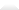Introduction to the Standard Template Library

The Standard Template Library, or STL, is a C++ library of container classes, algorithms, and iterators; it provides many of the basic algorithms and data structures of computer science. The STL is a generic library, meaning that its components are heavily parameterized: almost every component in the STL is a template. You should make sure that you understand how templates work in C++ before you use the STL.

Containers and algorithms

Like many class libraries, the STL includes container classes: classes whose purpose is to contain other objects. The STL includes the classes vector, list, deque, set, multiset, map, multimap, hash_set, hash_multiset, hash_map, and hash_multimap. Each of these classes is a template, and can be instantiated to contain any type of object. You can, for example, use a vector<int> in much the same way as you would use an ordinary C array, except that vector eliminates the chore of managing dynamic memory allocation by hand.

`vector<int> v(3); // Declare a vector of 3 elements.`

`v = 7;`

`v = v + 3;`

`v = v + v; // v == 7, v == 10, v == 17`

The STL also includes a large collection of algorithms that manipulate the data stored in containers. You can reverse the order of elements in a vector , for example, by using the reverse algorithm.

`reverse(v.begin(), v.end()); // v == 17, v == 10, v == 7`

There are two important points to notice about this call to reverse . First, it is a global function, not a member function. Second, it takes two arguments rather than one: it operates on a range of elements, rather than on a container. In this particular case the range happens to be the entire container v.

The reason for both of these facts is the same: reverse, like other STL algorithms, is decoupled from the STL container classes. This means that reverse can be used not only to reverse elements in vectors, but also to reverse elements in lists, and even elements in C arrays. The following program is also valid.

`double A = { 1.2, 1.3, 1.4, 1.5, 1.6, 1.7 };`

`reverse(A, A + 6);`

`for (int i = 0; i < 6; ++i) cout << 'A[' << i << '] = ' << A[i];`

This example uses a range , just like the example of reversing a vector: the first argument to reverse is a pointer to the beginning of the range, and the second argument points one element past the end of the range. This range is denoted [A, A + 6) ; the asymmetrical notation is a reminder that the two endpoints are different, that the first is the beginning of the range and the second is one past the end of the range.

Iterators

In the example of reversing a C array, the arguments to reverse are clearly of type double*. What are the arguments to reverse if you are reversing a vector, though, or a list? That is, what exactly does reverse declare its arguments to be, and what exactly do v.begin() and v.end() return?

The answer is that the arguments to reverse are iterators , which are a generalization of pointers. Pointers themselves are iterators, which is why it is possible to reverse the elements of a C array. Similarly, vector declares the nested types iterator and const_iterator. In the example above, the type returned by v.begin() and v.end() is vector<int>::iterator. There are also some iterators, such as istream_iterator and ostream_iterator, that aren't associated with containers at all.

Iterators are the mechanism that makes it possible to decouple algorithms from containers: algorithms are templates, and are parameterized by the type of iterator, so they are not restricted to a single type of container. Consider, for example, how to write an algorithm that performs linear search through a range. This is the STL's find algorithm.

`template <class InputIterator, class T>`

`InputIterator find(InputIterator first, InputIterator last, const T& value) {`

` while (first != last && *first != value) ++first;`

` return first;`

`}`

Find takes three arguments: two iterators that define a range, and a value to search for in that range. It examines each iterator in the range [first, last), proceeding from the beginning to the end, and stops either when it finds an iterator that points to value or when it reaches the end of the range.

First and last are declared to be of type InputIterator , and InputIterator is a template parameter. That is, there isn't actually any type called InputIterator: when you call find, the compiler substitutes the actual type of the arguments for the formal type parameters InputIterator and T. If the first two arguments to find are of type int* and the third is of type int , then it is as if you had called the following function.

`int* find(int* first, int* last, const int& value) {`

` while (first != last && *first != value) ++first;`

` return first;`

`}`

Concepts and Modeling

One very important question to ask about any template function, not just about STL algorithms, is what the set of types is that may correctly be substituted for the formal template parameters. Clearly, for example, int* or double* may be substituted for find 's formal template parameter InputIterator . Equally

Добавить отзыв
ВСЕ ОТЗЫВЫ О КНИГЕ В ИЗБРАННОЕ

0Вы можете отметить интересные вам фрагменты текста, которые будут доступны по уникальной ссылке в адресной строке браузера.

Отметить Добавить цитату